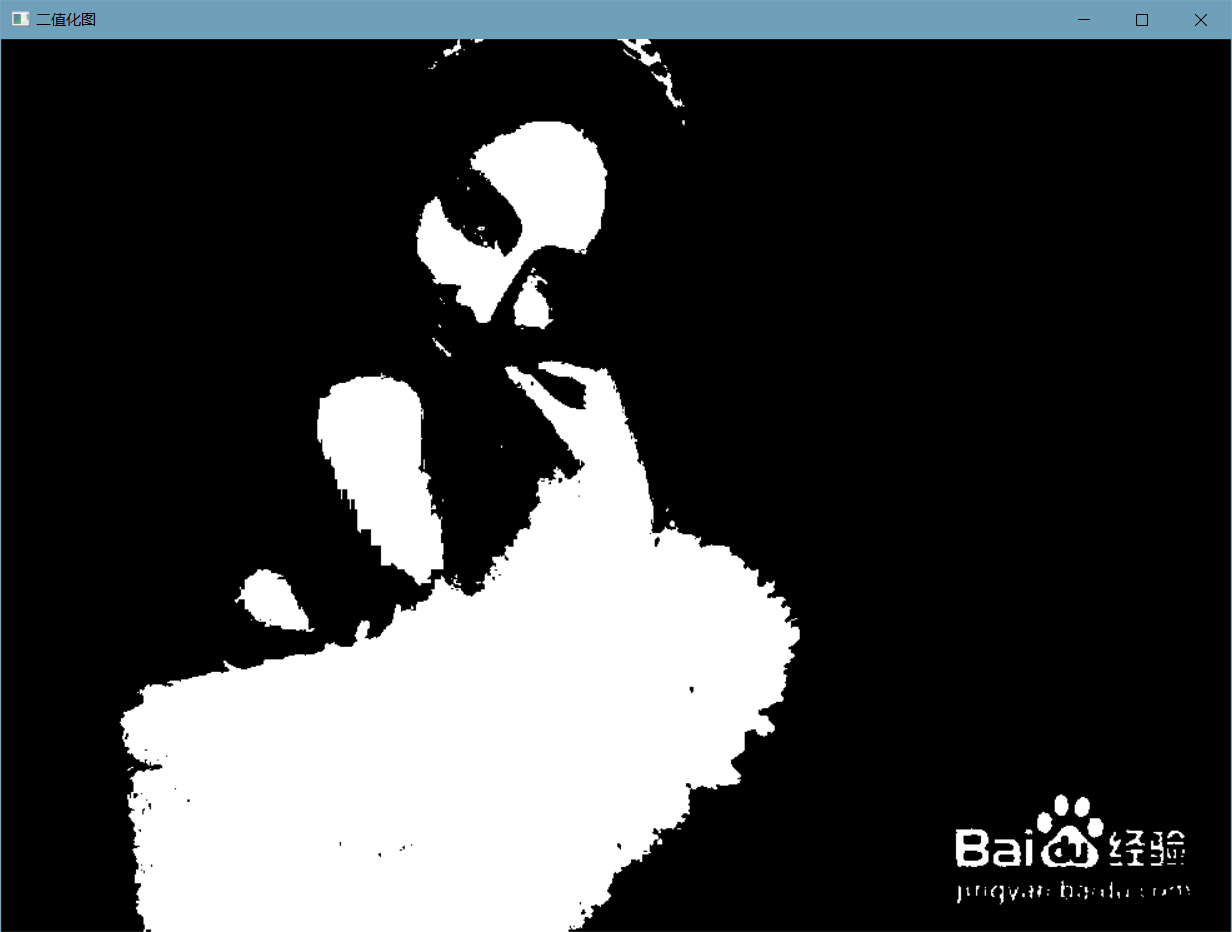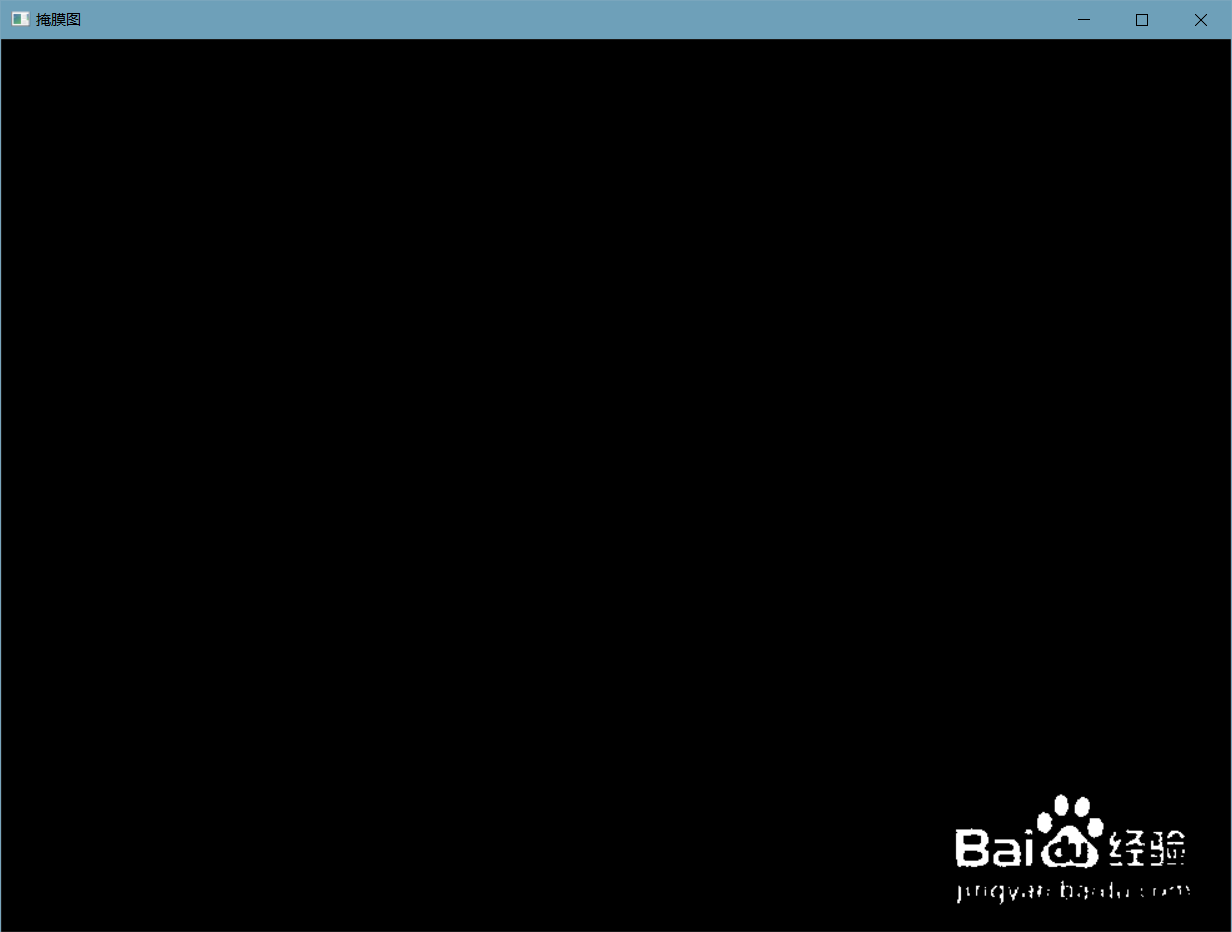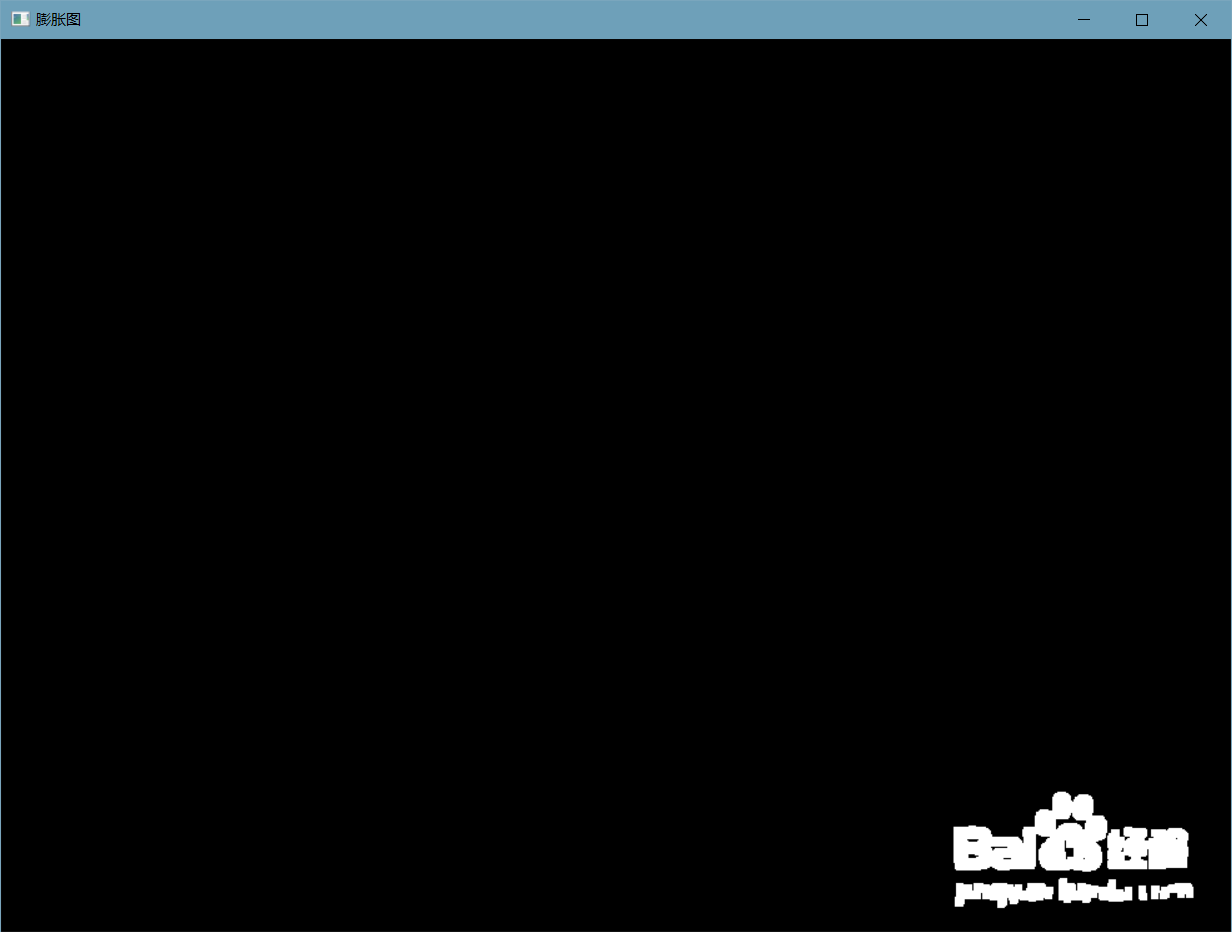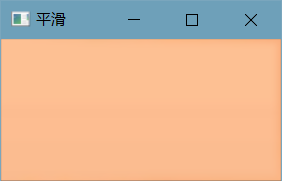# OpenCV数字图像处理实战一：去水印（C++）

## 1、简单版去水印

### 1.1 获取原图

`````` //  1. 获取原图
if (src.empty())
{
cout << "No Image!" << endl;
system("pause");
return -1;
}
imshow("原图", src);
``````### 1.2 灰度化

``````   //   2. 灰度化
Mat gray;
cvtColor(src, gray, COLOR_BGR2GRAY);
imshow("灰度图", gray);
``````### 1.3 二值化

``````//  3. 图像二值化，筛选出白色区域部分
Mat binary;
//  在这里使用图像的平均值作为阈值T
Scalar T = mean(gray);
threshold(gray, binary, 220, 255, THRESH_BINARY);
imshow("二值化图", binary);
``````### 1.4 生成掩膜图

`````` //  4.提取图片下方的水印，制作掩模图像
int start_x = 0.8 * src.rows;
int end_x = src.rows;
int start_y = 0.8 * src.cols -40;
int end_y = src.cols;

//遍历图像像素，提取出水印部分像素，制作掩模图像
for (int i = start_x; i < end_x; i++)
{
uchar* data = binary.ptr<uchar>(i);
for (int j = start_y; j < end_y; j++)
{
//cout << binary.ptr<uchar>(i)[j]<<" ";
if (data[j] == 255)
{
}

}
cout << endl;
}
``````### 1.5 膨胀

``````//  5.将掩模进行膨胀，使其能够覆盖图像更大区域
Mat kernel = getStructuringElement(MORPH_RECT, Size(6, 6));
````````````getStructuringElement(int shape, Size ksize, Point anchor)

getStructuringElement源码介绍看附录
``````

### 1.6 修复

``````//  6.使用inpaint进行图像修复
Mat result;
imshow("image show", result);
````````````void inpaint(
InputArray src,
OutputArray dst,
int flags );

``````

## 2、进阶版去水印

### 2.1 获取原图

``````//  1. 获取原图
if (dst.empty())
{
cout << "No Image!" << endl;
system("pause");
return -1;
}
``````### 2.2 通过鼠标定位水印位置

``````void on_mouse(int event, int x, int y, int flags, void* userdata) {
Mat dst = (*(Mat*)userdata).clone();
Mat src(*(Mat*)userdata);

char temp;
//判断左键按下，记录起始点坐标
if (event == EVENT_LBUTTONDOWN) {
point1.x = x;
point1.y = y;
}
else if (event == EVENT_MOUSEMOVE && (flags & EVENT_FLAG_LBUTTON)) {
//计算需画的矩形
//Rect rect(startP.x, startP.y, x - startP.x, y - startP.y);
//在图像上画出矩形
point2.x = x;
point2.y = y;

sprintf_s(temp, "(%d,%d)", x, y);//坐标
putText(dst, temp, point2, FONT_HERSHEY_SIMPLEX, 0.5, Scalar(0, 0, 0, 255));//实时显示鼠标移动的坐标
rectangle(dst, point1, Point(x, y), Scalar(250, 0, 0), 2, 0);
imshow("image show", dst);
}
//左键松开，在原图像画出矩形
else if (event == EVENT_LBUTTONUP) {
point2.x = x;
point2.y = y;

//Rect rect(point1.x, point1.y, x - point1.x, y - point1.y);
rectangle(dst, point1, point2, Scalar(0, 0, 250), 2, 0);
imshow("image show", dst);

}

}
``````

（1）按住左键进行移动，此时会显示当前坐标信息以及当前选中的区域（用蓝色标出）。（2）释放左键，表示已经选好要去水印的区域，此时用红色矩形框标出。### 2.3 使用周围像素进行替换

`````` //对选中区域进行像素替换，偏移3个像素，根据实际情况调节
for (int i = min(point1.y, point2.y); i < max(point2.y, point1.y); i++)
{
for (int j = min(point1.x, point2.x); j < max(point2.x, point1.x); j++)
{

src.at<Vec3b>(i, j) = src.at<Vec3b>(i, j - 3);
src.at<Vec3b>(i, j) = src.at<Vec3b>(i, j - 3);
src.at<Vec3b>(i, j) = src.at<Vec3b>(i, j - 3);

}
}

imshow("imagg", src);
``````### 2.4 对局部区域进行平滑处理

``````  //对选中区域周围进行平滑处理
Mat imageroi = src(Range(point1.y - 3, point2.y + 3), Range(point1.x - 3, point2.x + 3));
GaussianBlur(imageroi, imageroi, Size(15, 15), 0, 0);
``````### 2.5 将平滑结果粘贴到替换区域

``````Mat img1 = (src).clone();
Mat imgdst = img1(Rect(point1.x - 3,point1.y - 3, point2.x - point1.x, point2.y - point1.y));
imageroi.copyTo(imgdst);
``````### 2.6 美颜（非必须）

`````` //对整体图像双边滤波（对人像有美颜效果）
bilateralFilter(img1, dst, 15, 30, 5);
``````## 附录

getStructuringElement源码介绍

``````cv::Mat cv::getStructuringElement(int shape, Size ksize, Point anchor)
{
int i, j;
int r = 0, c = 0;
double inv_r2 = 0;

CV_Assert( shape == MORPH_RECT || shape == MORPH_CROSS || shape == MORPH_ELLIPSE );　　　　　　　　//目前支持三种形状的单元创建： 矩形， 十字形， 椭圆形；

anchor = normalizeAnchor(anchor, ksize);　　　　　　　　　　　　　　　　　　　　//当默认为-1，-1时， 计算anchor;

if( ksize == Size(1,1) )　　　　　　　　　　　　　　　　　　//当给定大小为1，1时，表明是一个点， 可以用矩形来表示；
shape = MORPH_RECT;

if( shape == MORPH_ELLIPSE )　　　　　　　　　　　　　　　//椭圆；
{
r = ksize.height/2;
c = ksize.width/2;
inv_r2 = r ? 1./((double)r*r) : 0;
}

Mat elem(ksize, CV_8U);

for( i = 0; i < ksize.height; i++ )　　　　　　　　　　　　　　　　　　　　//对每一行，计算0，1的范围；
{
uchar* ptr = elem.ptr(i);
int j1 = 0, j2 = 0;

if( shape == MORPH_RECT || (shape == MORPH_CROSS && i == anchor.y) )　　　　　　　　//矩形，或十字y锚点时  j2为ksize.width;
j2 = ksize.width;
else if( shape == MORPH_CROSS )
j1 = anchor.x, j2 = j1 + 1;
else　　　　　　　　　　　　　　　　　　　　　　　　　　　　　　　　　　　　　　　　　　　　　　　//椭圆；
{
int dy = i - r;
if( std::abs(dy) <= r )
{
int dx = saturate_cast<int>(c*std::sqrt((r*r - dy*dy)*inv_r2));　　　　　　　　//计算得到x的偏移；
j1 = std::max( c - dx, 0 );
j2 = std::min( c + dx + 1, ksize.width );
}
}

for( j = 0; j < j1; j++ )　　　　　　　　　　　　　　　　//从这三个for可以看出, (0,j1)之间为 0,  (j1, j2)之间为1,  (j2, ksize.width)之间为0;
ptr[j] = 0;
for( ; j < j2; j++ )
ptr[j] = 1;
for( ; j < ksize.width; j++ )
ptr[j] = 0;
}

return elem;
}
``````

### ubuntu平台下ffmpeg的编译安装方法_FISH_LJZ的博客-程序员资料

FFmpeg是一套可以用来记录、转换数字音视频，并能将其转化为流的开源计算机程序。它包括了目前领先的音/视频编码库libavcodec等。libavformat ：用于各种音视频封装格式的生成和解析，包括获取解码所需信息以生成解码上下文结构和读取音视频帧等功能； libavcodec ：用于各种类型声音/图像编解码； libavutil ：包含一些公共的工具函数； libsws

### win10修改ntp服务器地址,win10怎么设置ntp服务器地址_白头如新倾盖如故的博客-程序员资料

win10怎么设置ntp服务器地址 内容精选换一换以NTP服务器、DNS服务器的操作系统均为SUSE为例：登录Linux弹性云服务器。执行以下命令，切换至root用户。sudo su -sudo su -执行以下命令，编辑ntp.conf文件。vim /etc/ntp.confvim /etc/ntp.conf添加以下语句，配置NTP服务器。server NTP服务器域名或IP地址示例：有，该NT...

### 各个大数据相关框架启动与停止的命令_东心十的博客-程序员资料

zookeeper:三个节点上都执行： zkServer.sh start 启动单个节点的zk服务查看zk进程是否存在：jps ，发现一个进程 QuorumPeerMainzkServer.sh status 查看该zk服务器是follower还是leader。hdfs:start-dfs.shstop-dfs.sh查看hdfs进程是否存在：jpsbin/hdfs zk...